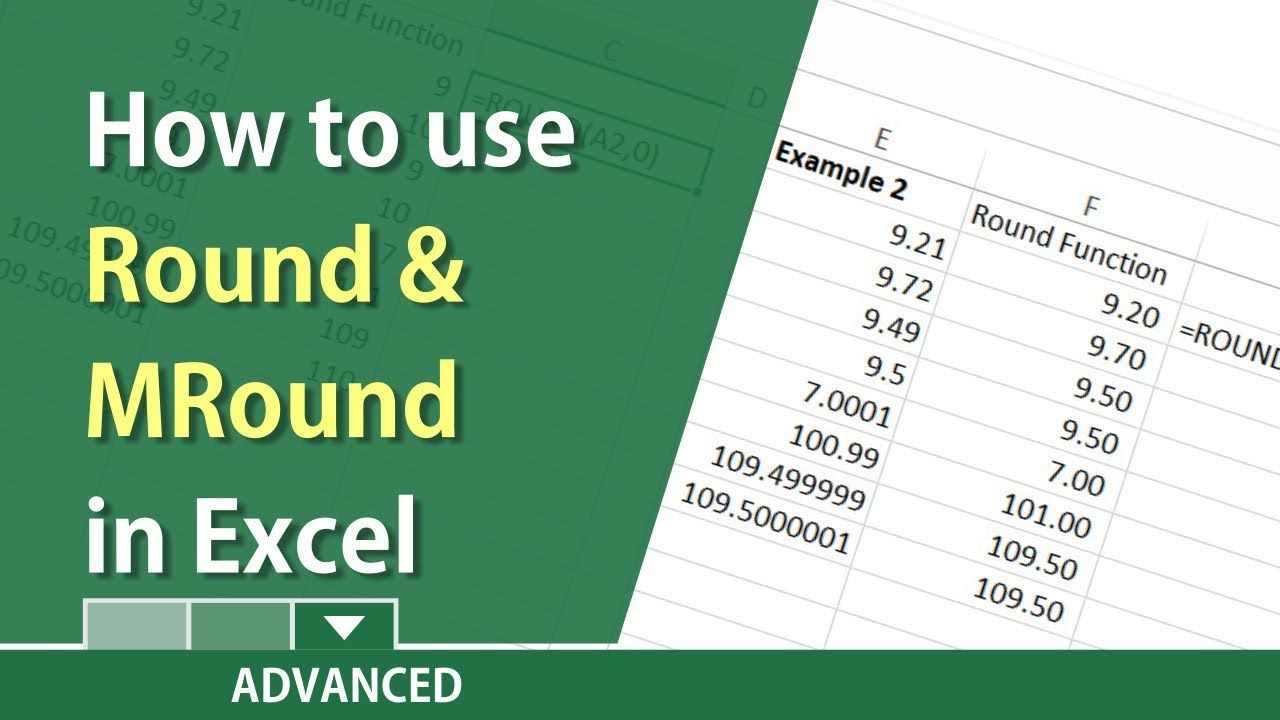# Excel – Round and MRound Functions in Excel by Chris Menard

Video on ROUNDUP Function

The ROUND function rounds a number to a specified number of digits.

TimeStamps:
00:15 Round 0 decimal place
01:30 Formula Text Function
01:40 Round 1 decimal place
02:15 Round 0 and subtract .01
03:21Round -1
04:40 MRound function used for Timekeeping

Examples:

A1 contains 34.7725, and you want to round that value to two decimal places, you can use the following formula:

=ROUND(A1, 2) The result will be 34.77

A1 contains 34.7725, and you want to round that value to one decimal places, you can use the following formula:

=Round(A1,1)

The result of this function is 34.8

9.42 Rounded one decimal place will be 9.4.
9.46 Rounded one decimal place will be 9.5

The round function has two arguments. The cell reference and the number of decimal places you want to round.

To end all numbers in .99, example the number 23.00, to show .99 would be 22.99. Use the round function and subtract .01. Example: =Round(A1,0)-.01 Be sure to format the cells for two decimal places to see the result.

The MRound function rounds up, away from zero, if the remainder of dividing a number by multiple is greater than or equal to half the value of multiple. Use the MRound function for the 7-minute rule for time clocks. Many employers use a 15-minute clock. Clocking in at 7:07 AM is clocking in at 7:00 AM. Clocking in at 7:08 AM is clocking in at 7:15 AM.

The DOL allows employers to use the 7-minute rule for hourly or non-exempt employees.
DOL Article about the 7-minute rule

Chris Menard -Website

Microsoft Support Page for Round:

Nguồn: https://cinausp.org

Xem thêm bài viết khác: https://cinausp.org/tong-hop

## 2 thoughts on “Excel – Round and MRound Functions in Excel by Chris Menard”

1.Ivonna Ivie says:

Hello @ChrisMenard I don't see the tutorial on roundup and rounddown on Nov 1, 2018, did I miss it?

2.Ivonna Ivie says:

Thank you! I messed around with Excel trying to get .99 for hours yesterday!